Tamil Nadu Board of Secondary EducationSSLC (English Medium) Class 9th
Advertisement Remove all ads

# Samacheer Kalvi solutions for Mathematics Class 9th Tamil Nadu State Board chapter 3 - Algebra [Latest edition]

Advertisement Remove all ads

#### Chapters## Chapter 3: Algebra

Exercise 3.1Exercise 3.2Exercise 3.3Exercise 3.4Exercise 3.5Exercise 3.6Exercise 3.7Exercise 3.8Exercise 3.9Exercise 3.10Exercise 3.11Exercise 3.12Exercise 3.13Exercise 3.14Exercise 3.15
Exercise 3.1 [Pages 87 - 88]

### Samacheer Kalvi solutions for Mathematics Class 9th Tamil Nadu State Board Chapter 3 Algebra Exercise 3.1 [Pages 87 - 88]

Exercise 3.1 | Q 1. (i) | Page 87

Identify the following expression is polynomial. If not give reason:

1/x^2 + 3x - 4

Exercise 3.1 | Q 1. (ii) | Page 87

Identify the following expression is polynomial. If not give reason:

x2(x – 1)

Exercise 3.1 | Q 1. (iii) | Page 87

Identify the following expression is polynomial. If not give reason:

1/x(x + 5)

Exercise 3.1 | Q 1. (iv) | Page 87

Identify the following expression is polynomial. If not give reason:

1/(x^(-2)) + 1/(x^(-1)) + 7

Exercise 3.1 | Q 1. (v) | Page 87

Identify the following expression is polynomial. If not give reason:

sqrt(5)x^2 + sqrt(3)x + sqrt(2)

Exercise 3.1 | Q 1. (vi) | Page 87

Identify the following expression is polynomial. If not give reason:

"m"^2 - root(3)("m") + 7"m" - 10

Exercise 3.1 | Q 2. (i) | Page 87

Write the coefficient of x2 and x in the following polynomials

4 + 2/5x^2 - 3x

Exercise 3.1 | Q 2. (ii) | Page 87

Write the coefficient of x2 and x in the following polynomials

6 - 2x^2 + 3x^3 - sqrt(7)x

Exercise 3.1 | Q 2. (iii) | Page 87

Write the coefficient of x2 and x in the following polynomials

πx2 – x + 2

Exercise 3.1 | Q 2. (iv) | Page 87

Write the coefficient of x2 and x in the following polynomials

sqrt(3)x^2 + sqrt(2)x + 0.5

Exercise 3.1 | Q 2. (v) | Page 87

Write the coefficient of x2 and x in the following polynomials

x^2 - 7/2 x + 8

Exercise 3.1 | Q 2. (v) | Page 87

Find the degree of the following polynomial

2sqrt(5)"p"^4 - (8"p"^3)/sqrt(3) + (2"p"^2)/7

Exercise 3.1 | Q 3. (i) | Page 87

Find the degree of the following polynomial

1 - sqrt(2)y^2 + y^7

Exercise 3.1 | Q 3. (ii) | Page 87

Find the degree of the following polynomial

(x^3 - x^4 + 6x^6)/x^2

Exercise 3.1 | Q 3. (iii) | Page 87

Find the degree of the following polynomial

x3(x2 + x)

Exercise 3.1 | Q 3. (iv) | Page 87

Find the degree of the following polynomial

3x4 + 9x2 + 27x

Exercise 3.1 | Q 4. (i) | Page 87

Rewrite the following polynomial in standard form

x - 9 + sqrt(7)x^3 + 6x^2

Exercise 3.1 | Q 4. (ii) | Page 87

Rewrite the following polynomial in standard form

sqrt(2)x^2 - 7/2 x^4 + x - 5x^3

Exercise 3.1 | Q 4. (iii) | Page 87

Rewrite the following polynomial in standard form

7x^3 - 6/5x^2 + 4x - 1

Exercise 3.1 | Q 4. (iv) | Page 87

Rewrite the following polynomial in standard form

y^2 + sqrt(5)y^3 - 11 - 7/3y + 9y^4

Exercise 3.1 | Q 5. (i) | Page 87

Add the following polynomials and find the degree of the resultant polynomial

p(x) = 6x2 – 7x + 2, q(x) = 6x3 – 7x + 15

Exercise 3.1 | Q 5. (ii) | Page 87

Add the following polynomials and find the degree of the resultant polynomial

h(x) = 7x3 – 6x + 1, f(x) = 7x2 + 17x – 9

Exercise 3.1 | Q 5. (iii) | Page 87

Add the following polynomials and find the degree of the resultant polynomial

f(x) = 16x4 – 5x2 + 9, g(x) = −6x3 + 7x – 15

Exercise 3.1 | Q 6. (i) | Page 87

Subtract the second polynomial from the first polynomial and find the degree of the resultant polynomial

p(x) = 7x2 + 6x – 1, q(x) = 6x – 9

Exercise 3.1 | Q 6. (ii) | Page 88

Subtract the second polynomial from the first polynomial and find the degree of the resultant polynomial

f(y) = 6y2 – 7y + 2, g(y) = 7y + y3

Exercise 3.1 | Q 6. (iii) | Page 88

Subtract the second polynomial from the first polynomial and find the degree of the resultant polynomial

h(z) = z5 – 6z4 + z, f(z) = 6z2 + 10z – 7

Exercise 3.1 | Q 7 | Page 88

What should be added to 2x3 + 6x2 – 5x + 8 to get 3x3 – 2x2 + 6x + 15?

Exercise 3.1 | Q 8 | Page 88

What must be subtracted from 2x4 + 4x2 – 3x + 7 to get 3x3 – x2 + 2x + 1?

Exercise 3.1 | Q 9. (i) | Page 88

Multiply the following polynomials and find the degree of the resultant polynomial:

p(x) = x2 – 9, q(x) = 6x2 + 7x – 2

Exercise 3.1 | Q 9. (ii) | Page 88

Multiply the following polynomials and find the degree of the resultant polynomial:

f(x) = 7x + 2, g(x) = 15x – 9

Exercise 3.1 | Q 9. (iii) | Page 88

Multiply the following polynomials and find the degree of the resultant polynomial:

h(x) = 6x2 – 7x + 1, f(x) = 5x – 7

Exercise 3.1 | Q 10 | Page 88

The cost of a chocolate is Rs. (x + y) and Amir bought (x + y) chocolates. Find the total amount paid by him in terms of x and y. If x = 10, y = 5 find the amount paid by him

Exercise 3.1 | Q 11 | Page 88

The length of a rectangle is (3x + 2) units and it’s breadth is (3x – 2) units. Find its area in terms of x. What will be the area if x = 20 units

Exercise 3.1 | Q 12 | Page 88

p(x) is a polynomial of degree 1 and q(x) is a polynomial of degree 2. What kind of the polynomial is p(x) × q(x)?

Advertisement Remove all ads
Exercise 3.2 [Pages 91 - 92]

### Samacheer Kalvi solutions for Mathematics Class 9th Tamil Nadu State Board Chapter 3 Algebra Exercise 3.2 [Pages 91 - 92]

Exercise 3.2 | Q 1. (i) | Page 91

Find the value of the polynomial f(y) = 6y – 3y2 + 3 at y = 1

Exercise 3.2 | Q 1. (ii) | Page 91

Find the value of the polynomial f(y) = 6y – 3y2 + 3 at y = –1

Exercise 3.2 | Q 1. (iii) | Page 91

Find the value of the polynomial f(y) = 6y – 3y2 + 3 at y = 0

Exercise 3.2 | Q 2 | Page 91

If p(x) = x^2 - 2sqrt(2)x + 1, find "p"(2sqrt(2))

Exercise 3.2 | Q 3. (i) | Page 91

Find the zero of the polynomial of the following:

p(x) = x – 3

Exercise 3.2 | Q 3. (ii) | Page 91

Find the zero of the polynomial of the following:

p(x) = 2x + 5

Exercise 3.2 | Q 3. (iii) | Page 91

Find the zero of the polynomial of the following:

q(y) = 2y – 3

Exercise 3.2 | Q 3. (iv) | Page 91

Find the zero of the polynomial of the following :

f(z) = 8z

Exercise 3.2 | Q 3. (iv) | Page 91

Find the zero of the polynomial in the following:

h(x) = ax + b, a ≠ 0, a, b ∈ R

Exercise 3.2 | Q 3. (v) | Page 91

Find the zero of the polynomial of the following:

p(x) = ax when a ≠ 0

Exercise 3.2 | Q 4. (i) | Page 91

Find the roots of the polynomial equation

5x – 6 = 0

Exercise 3.2 | Q 4. (ii) | Page 91

Find the roots of the polynomial equation

x + 3 = 0

Exercise 3.2 | Q 4. (iii) | Page 91

Find the roots of the polynomial equation

10x + 9 = 0

Exercise 3.2 | Q 4. (iv) | Page 91

Find the roots of the polynomial equation

9x – 4 = 0

Exercise 3.2 | Q 5. (i) | Page 91

Verify whether the following are zeros of the polynomial indicated against them, or not

p(x) = 2x − 1, x = 1/2

Exercise 3.2 | Q 5. (ii) | Page 91

Verify whether the following are zeros of the polynomial, indicated against them, or not

p(x) = x3 – 1, x = 1

Exercise 3.2 | Q 5. (iii) | Page 91

Verify whether the following are zeros of the polynomial, indicated against them, or not

p(x) = ax + b, x = (-"b")/"a"

Exercise 3.2 | Q 5. (iv) | Page 91

Verify whether the following are zeros of the polynomial, indicated against them, or not

p(x) = (x + 3) (x – 4), x = −3, x = 4

Exercise 3.2 | Q 6. (i) | Page 92

Find the number of zeros of the following polynomial represented by their graph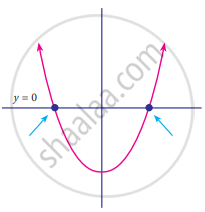Exercise 3.2 | Q 6. (ii) | Page 92

Find the number of zeros of the following polynomial represented by their graph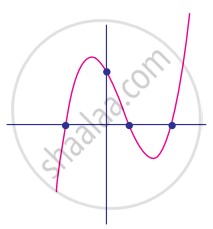Exercise 3.2 | Q 6. (iii) | Page 92

Find the number of zeros of the following polynomial represented by their graph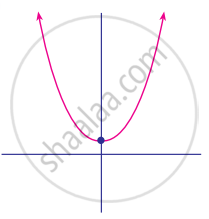Exercise 3.2 | Q 6. (iv) | Page 92

Find the number of zeros of the following polynomial represented by their graph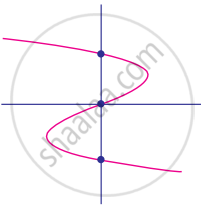Exercise 3.2 | Q 6. (v) | Page 92

Find the number of zeros of the following polynomial represented by their graph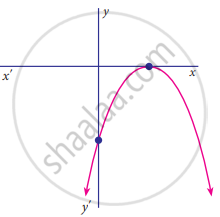Advertisement Remove all ads
Exercise 3.3 [Pages 96 - 97]

### Samacheer Kalvi solutions for Mathematics Class 9th Tamil Nadu State Board Chapter 3 Algebra Exercise 3.3 [Pages 96 - 97]

Exercise 3.3 | Q 1 | Page 96

Check whether p(x) is a multiple of g(x) or not

p(x) = x3 – 5x2 + 4x – 3, g(x) = x – 2

Exercise 3.3 | Q 2. (i) | Page 96

By remainder theorem, find the remainder when, p(x) is divided by g(x) where, p(x) = x3 – 2x2 – 4x – 1; g(x) = x + 1

Exercise 3.3 | Q 2. (ii) | Page 96

By remainder theorem, find the remainder when, p(x) is divided by g(x) where, p(x) = 4x3 – 12x2 + 14x – 3; g(x) = 2x – 1

Exercise 3.3 | Q 2. (iii) | Page 96

By remainder theorem, find the remainder when, p(x) is divided by g(x) where, p(x) = x3 – 3x2 + 4x + 50; g(x) = x – 3

Exercise 3.3 | Q 3 | Page 96

Find the remainder when 3x3 – 4x2 + 7x – 5 is divided by (x + 3)

Exercise 3.3 | Q 4 | Page 96

What is the remainder when x2018 + 2018 is divided by x – 1

Exercise 3.3 | Q 5 | Page 96

For what value of k is the polynomial p(x) = 2x3 – kx2 + 3x + 10 exactly divisible by (x – 2)

Exercise 3.3 | Q 6 | Page 96

If two polynomials 2x3 + ax2 + 4x – 12 and x3 + x2 – 2x + a leave the same remainder when divided by (x – 3), find the value of a and also find the remainder.

Exercise 3.3 | Q 7. (i) | Page 96

Determine whether (x – 1) is a factor of the following polynomials:

x3 + 5x2 – 10x + 4

Exercise 3.3 | Q 7. (ii) | Page 96

Determine whether (x – 1) is a factor of the following polynomials:

x4 + 5x2 – 5x + 1

Exercise 3.3 | Q 8 | Page 97

Using factor theorem, show that (x – 5) is a factor of the polynomial

2x3 – 5x2 – 28x + 15

Exercise 3.3 | Q 9 | Page 97

Determine the value of m, if (x + 3) is a factor of x3 – 3x2 – mx + 24

Exercise 3.3 | Q 10 | Page 97

If both (x − 2) and (x - 1/2) is the factors of ax2 + 5x + b, then show that a = b

Exercise 3.3 | Q 11 | Page 97

If (x – 1) divides the polynomial kx3 – 2x2 + 25x – 26 without remainder, then find the value of k

Exercise 3.3 | Q 12 | Page 97

Check if (x + 2) and (x – 4) are the sides of a rectangle whose area is x2 – 2x – 8 by using factor theorem

Advertisement Remove all ads
Exercise 3.4 [Pages 101 - 102]

### Samacheer Kalvi solutions for Mathematics Class 9th Tamil Nadu State Board Chapter 3 Algebra Exercise 3.4 [Pages 101 - 102]

Exercise 3.4 | Q 1. (i) | Page 101

Expand the following:

(2x + 3y + 4z)

Exercise 3.4 | Q 1. (ii) | Page 101

Expand the following:

(−p + 2q + 3r)2

Exercise 3.4 | Q 1. (iii) | Page 101

Expand the following:

(2p + 3)(2p – 4)(2p – 5)

Exercise 3.4 | Q 1. (iv) | Page 101

Expand the following:

(3a + 1)(3a – 2)(3a + 4)

Exercise 3.4 | Q 2. (i) | Page 101

Using algebraic identity, find the coefficients of x2, x and constant term without actual expansion

(x + 5)(x + 6)(x + 7)

Exercise 3.4 | Q 2. (ii) | Page 101

Using algebraic identity, find the coefficients of x2, x and constant term without actual expansion

(2x + 3)(2x – 5)(2x – 6)

Exercise 3.4 | Q 3. (i) | Page 101

If (x + a)(x + b)(x + c) = x3 + 14x2 + 59x + 70, find the value of a + b + c

Exercise 3.4 | Q 3. (ii) | Page 101

If (x + a)(x + b)(x + c) = x3 + 14x2 + 59x + 70, find the value of 1/"a" + 1/"b" + 1/"c"

Exercise 3.4 | Q 3. (iii) | Page 101

If (x + a)(x + b)(x + c) = x3 + 14x2 + 59x + 70, find the value of a2 + b2 + c

Exercise 3.4 | Q 3. (iv) | Page 101

If (x + a)(x + b)(x + c) = x3 + 14x2 + 59x + 70, find the value of "a"/"bc" + "b"/"ac" + "c"/"ab"

Exercise 3.4 | Q 4. (i) | Page 101

Expand: (3a – 4b)

Exercise 3.4 | Q 4. (ii) | Page 101

Expand: [x + 1/y]^3

Exercise 3.4 | Q 5. (i) | Page 101

Evaluate the following by using identities:

983

Exercise 3.4 | Q 5. (ii) | Page 101

Evaluate the following by using identities:

10013

Exercise 3.4 | Q 6 | Page 101

If (x + y + z) = 9 and (xy + yz + zx) = 26, then find the value of x2 + y2 + z2

Exercise 3.4 | Q 7 | Page 101

Find 27a3 + 64b3, if 3a + 4b = 10 and ab = 2

Exercise 3.4 | Q 8 | Page 101

Find x3 – y3, if x – y = 5 and xy = 14.

Exercise 3.4 | Q 9 | Page 101

If "a" + 1/"a" = 6, then find the value of "a"^3 + 1/"a"^3

Exercise 3.4 | Q 10 | Page 101

If x^2 + 1/x^2 = 23, then find the value of x + 1/x and x^3 + 1/x^3

Exercise 3.4 | Q 11 | Page 101

If (y - 1/y)^3 = 27, then find the value of y^3 - 1/y^3

Exercise 3.4 | Q 12. (ii) | Page 102

Simplify: (2a + 3b + 4c) (4a2 + 9b2 + 16c2 – 6ab – 12bc – 8ca)

Exercise 3.4 | Q 12. (ii) | Page 102

Simplify: (x – 2y + 3z) (x2 + 4y2 + 9z2 + 2xy + 6yz – 3xz)

Exercise 3.4 | Q 13. (i) | Page 102

By using identity evaluate the following:

73 – 103 + 3

Exercise 3.4 | Q 13. (ii) | Page 102

By using identity evaluate the following:

1 + 1/8 - 27/8

Exercise 3.4 | Q 14 | Page 102

If 2x – 3y – 4z = 0, then find 8x3 – 27y3 – 64z

Advertisement Remove all ads
Exercise 3.5 [Page 105]

### Samacheer Kalvi solutions for Mathematics Class 9th Tamil Nadu State Board Chapter 3 Algebra Exercise 3.5 [Page 105]

Exercise 3.5 | Q 1. (i) | Page 105

Factorise the following expression:

2a² + 4a²b + 8a²c

Exercise 3.5 | Q 1. (ii) | Page 105

Factorise the following expression:

ab – ac – mb + mc

Exercise 3.5 | Q 2. (i) | Page 105

Factorise the following:

x² + 4x + 4

Exercise 3.5 | Q 2. (ii) | Page 105

Factorise the following:

3a² – 24ab + 48b²

Exercise 3.5 | Q 2. (iii) | Page 105

Factorise the following:

x5 – 16x

Exercise 3.5 | Q 2. (iv) | Page 105

Factorise the following:

"m"^2 + 1/"m"^2 - 23

Exercise 3.5 | Q 2. (v) | Page 105

Factorise the following:

6 – 216x

Exercise 3.5 | Q 2. (iv) | Page 105

Factorise the following:

"a"^2 + 1/"a"^2 - 18

Exercise 3.5 | Q 3. (i) | Page 105

Factorise the following:

4x2 + 9y2 + 25z2 + 12xy + 30yz + 20xz

Exercise 3.5 | Q 3. (ii) | Page 105

Factorise the following:

25x2 + 4y2 + 9z2 – 20xy + 12yz – 30xz

Exercise 3.5 | Q 4. (i) | Page 105

Factorise the following:

8x3 + 125y3

Exercise 3.5 | Q 4. (ii) | Page 105

Factorise the following:

27x3 – 8y3

Exercise 3.5 | Q 4. (iii) | Page 105

Factorise the following:

a6 – 64

Exercise 3.5 | Q 5. (i) | Page 105

Factorise the following:

x3 + 8y3 + 6xy – 1

Exercise 3.5 | Q 5. (ii) | Page 105

Factorise the following:

l3 – 8m3 – 27n3 – 18lmn

Advertisement Remove all ads
Exercise 3.6 [Page 107]

### Samacheer Kalvi solutions for Mathematics Class 9th Tamil Nadu State Board Chapter 3 Algebra Exercise 3.6 [Page 107]

Exercise 3.6 | Q 1. (i) | Page 107

Factorise the following:

x² + 10x + 24

Exercise 3.6 | Q 1. (ii) | Page 107

Factorise the following:

z² + 4z – 12

Exercise 3.6 | Q 1. (iii) | Page 107

Factorise the following:

p² – 6p – 16

Exercise 3.6 | Q 1. (iv) | Page 107

Factorise the following:

t² + 72 – 17t

Exercise 3.6 | Q 1. (v) | Page 107

Factorise the following:

y2 – 16y – 80

Exercise 3.6 | Q 1. (vi) | Page 107

Factorise the following:

a2 + 10a – 600

Exercise 3.6 | Q 2. (i) | Page 107

Factorise the following:

2a2 + 9a + 10

Exercise 3.6 | Q 2. (ii) | Page 107

Factorise the following:

5x2 – 29xy – 42y2

Exercise 3.6 | Q 2. (iii) | Page 107

Factorise the following:

9 – 18x + 8x2

Exercise 3.6 | Q 2. (iv) | Page 107

Factorise the following:

6x2 + 16xy + 8y2

Exercise 3.6 | Q 2. (v) | Page 107

Factorise the following:

12x2 + 36x2y + 27y2x2

Exercise 3.6 | Q 2. (vi) | Page 107

Factorise the following:

(a + b)2 + 9(a + b) + 18

Exercise 3.6 | Q 3. (i) | Page 107

Factorise the following:

(p – q)2 – 6(p – q) – 16

Exercise 3.6 | Q 3. (ii) | Page 107

Factorise the following:

m2 + 2mn – 24n2

Exercise 3.6 | Q 3. (iii) | Page 107

Factorise the following:

sqrt(5)"a"^2 + 2"a" - 3sqrt(5)

Exercise 3.6 | Q 3. (iv) | Page 107

Factorise the following:

a4 – 3a2 + 2

Exercise 3.6 | Q 3. (v) | Page 107

Factorise the following:

8m3 – 2m2n – 15mn2

Exercise 3.6 | Q 3. (vi) | Page 107

Factorise the following:

1/x^2 + 1/y^2 + 2/(xy)

Advertisement Remove all ads
Exercise 3.7 [Pages 111 - 112]

### Samacheer Kalvi solutions for Mathematics Class 9th Tamil Nadu State Board Chapter 3 Algebra Exercise 3.7 [Pages 111 - 112]

Exercise 3.7 | Q 1. (i) | Page 111

Find the quotient and remainder of the following.

(4x3 + 6x2 – 23x + 18) ÷ (x + 3)

Exercise 3.7 | Q 1. (ii) | Page 111

Find the quotient and remainder of the following.

(8y3 – 16y2 + 16y – 15) ÷ (2y – 1)

Exercise 3.7 | Q 1. (iii) | Page 111

Find the quotient and remainder of the following.

(8x3 – 1) ÷ (2x – 1)

Exercise 3.7 | Q 1. (iv) | Page 111

Find the quotient and remainder of the following.

(−18z + 14z2 + 24z3 + 18) ÷ (3z + 4)

Exercise 3.7 | Q 2 | Page 111

The area of a rectangle is x2 + 7x + 12. If its breadth is (x + 3), then find its length

Exercise 3.7 | Q 3 | Page 111

The base of a parallelogram is (5x + 4). Find its height if the area is 25x2 – 16

Exercise 3.7 | Q 4 | Page 111

The sum of (x + 5) observations is (x3 + 125). Find the mean of the observations

Exercise 3.7 | Q 5. (i) | Page 111

Find the quotient and remainder for the following using synthetic division:

(x3 + x2 – 7x – 3) ÷ (x – 3)

Exercise 3.7 | Q 5. (ii) | Page 111

Find the quotient and remainder for the following using synthetic division:

(x3 + 2x2 – x – 4) ÷ (x + 2)

Exercise 3.7 | Q 5. (iii) | Page 111

Find the quotient and remainder for the following using synthetic division:

(3x3 – 2x2 + 7x – 5) ÷ (x + 3)

Exercise 3.7 | Q 5. (iv) | Page 111

Find the quotient and remainder for the following using synthetic division:

(8x4 – 2x2 + 6x + 5) ÷ (4x + 1)

Exercise 3.7 | Q 6 | Page 111

If the quotient obtained on dividing (8x4 – 2x2 + 6x – 7) by (2x + 1) is (4x3 + px2 – qx + 3), then find p, q and also the remainder

Exercise 3.7 | Q 7 | Page 112

If the quotient obtained on dividing 3x3 + 11x2 + 34x + 106 by x – 3 is 3x2 + ax + b, then find a, b and also the remainder

Advertisement Remove all ads
Exercise 3.8 [Page 114]

### Samacheer Kalvi solutions for Mathematics Class 9th Tamil Nadu State Board Chapter 3 Algebra Exercise 3.8 [Page 114]

Exercise 3.8 | Q 1. (i) | Page 114

Factorise the following polynomials using synthetic division:

x3 – 3x2 – 10x + 24

Exercise 3.8 | Q 1. (ii) | Page 114

Factorise the following polynomials using synthetic division:

2x3 – 3x2 – 3x + 2

Exercise 3.8 | Q 1. (iii) | Page 114

Factorise the following polynomials using synthetic division:

– 7x + 3 + 4x3

Exercise 3.8 | Q 1. (iv) | Page 114

Factorise the following polynomials using synthetic division:

x3 + x2 – 14x – 24

Exercise 3.8 | Q 1. (v) | Page 114

Factorise the following polynomials using synthetic division:

x3 – 7x + 6

Exercise 3.8 | Q 1. (vi) | Page 114

Factorise the following polynomials using synthetic division:

x3 – 10x2 – x + 10

Advertisement Remove all ads
Exercise 3.9 [Page 115]

### Samacheer Kalvi solutions for Mathematics Class 9th Tamil Nadu State Board Chapter 3 Algebra Exercise 3.9 [Page 115]

Exercise 3.9 | Q 1. (i) | Page 115

Find the G.C.D for the following:

P5, P11, P9

Exercise 3.9 | Q 1. (ii) | Page 115

Find the G.C.D for the following:

4x3, y3, z3

Exercise 3.9 | Q 1. (iii) | Page 115

Find the G.C.D for the following:

9a2b2c3, 15a3b2c4

Exercise 3.9 | Q 1. (iv) | Page 115

Find the G.C.D for the following:

64x8, 240x6

Exercise 3.9 | Q 1. (v) | Page 115

Find the G.C.D for the following:

ab2c3, a2b3c, a3bc2

Exercise 3.9 | Q 1. (vi) | Page 115

Find the G.C.D for the following:

35x5y3z4, 49x2yz3, 14xy2z2

Exercise 3.9 | Q 1. (vii) | Page 115

Find the G.C.D for the following:

25ab3c, 100a2bc, 125ab

Exercise 3.9 | Q 1. (viii) | Page 115

Find the G.C.D for the following:

3abc, 5xyz, 7pqr

Exercise 3.9 | Q 2. (i) | Page 115

Find the G.C.D of the following:

(2x + 5), (5x + 2)

Exercise 3.9 | Q 2. (ii) | Page 115

Find the G.C.D of the following:

am+1, am+2, am+3

Exercise 3.9 | Q 2. (iii) | Page 115

Find the G.C.D of the following:

2a2 + a, 4a2 – 1

Exercise 3.9 | Q 2. (iv) | Page 115

Find the G.C.D of the following:

3a2, 5b3, 7c

Exercise 3.9 | Q 2. (v) | Page 115

Find the G.C.D of the following:

x4 – 1, x2 – 1

Exercise 3.9 | Q 2. (vi) | Page 115

Find the G.C.D of the following:

a3 – 9ax2, (a – 3x)2

Advertisement Remove all ads
Exercise 3.10 [Page 124]

### Samacheer Kalvi solutions for Mathematics Class 9th Tamil Nadu State Board Chapter 3 Algebra Exercise 3.10 [Page 124]

Exercise 3.10 | Q 1. (i) | Page 124

Draw the graph for the following

y = 2x

Exercise 3.10 | Q 1. (ii) | Page 124

Draw the graph for the following

y = 4x – 1

Exercise 3.10 | Q 1. (iii) | Page 124

Draw the graph for the following

y = (3/2)x + 3

Exercise 3.10 | Q 1. (iv) | Page 124

Draw the graph for the following

3x + 2y = 14

Exercise 3.10 | Q 2. (i) | Page 124

Solve graphically

x + y = 7, x – y = 3

Exercise 3.10 | Q 2. (ii) | Page 124

Solve graphically

3x + 2y = 4, 9x + 6y – 12 = 0

Exercise 3.10 | Q 2. (iii) | Page 124

Solve graphically

x/2 + y/4 = 1, x/2 + y/4 = 2

Exercise 3.10 | Q 2. (iv) | Page 124

Solve graphically

x – y = 0, y + 3 = 0

Exercise 3.10 | Q 2. (v) | Page 124

Solve graphically

y = 2x + 1, y + 3x – 6 = 0

Exercise 3.10 | Q 2. (vi) | Page 124

Solve graphically

x = −3, y = 3

Exercise 3.10 | Q 3 | Page 124

Two cars are 100 miles apart. If they drive towards each other they will meet in 1 hour. If they drive in the same direction they will meet in 2 hours. Find their speed by using graphical method.

Advertisement Remove all ads
Exercise 3.11 [Page 126]

### Samacheer Kalvi solutions for Mathematics Class 9th Tamil Nadu State Board Chapter 3 Algebra Exercise 3.11 [Page 126]

Exercise 3.11 | Q 1. (i) | Page 126

Solve, using the method of substitution

2x – 3y = 7, 5x + y = 9

Exercise 3.11 | Q 1. (ii) | Page 126

Solve, using the method of substitution

1.5x + 0.1y = 6.2, 3x – 0.4y = 11.2

Exercise 3.11 | Q 1. (iii) | Page 126

Solve, using the method of substitution

10% of x + 20% of y = 24, 3x – y = 20

Exercise 3.11 | Q 1. (iv) | Page 126

Solve, using the method of substitution

sqrt(2)x - sqrt(3)y = 1, sqrt(3)x - sqrt(8)y = 0

Exercise 3.11 | Q 2 | Page 126

Raman’s age is three times the sum of the ages of his two sons. After 5 years his age will be twice the sum of the ages of his two sons. Find the age of Raman.

Exercise 3.11 | Q 3 | Page 126

The middle digit of a number between 100 and 1000 is zero and the sum of the other digit is 13. If the digits are reversed, the number so formed exceeds the original number by 495. Find the number

Advertisement Remove all ads
Exercise 3.12 [Page 128]

### Samacheer Kalvi solutions for Mathematics Class 9th Tamil Nadu State Board Chapter 3 Algebra Exercise 3.12 [Page 128]

Exercise 3.12 | Q 1. (i) | Page 128

Solve by the method of elimination

2x – y = 3, 3x + y = 7

Exercise 3.12 | Q 1. (ii) | Page 128

Solve by the method of elimination

x – y = 5, 3x + 2y = 25

Exercise 3.12 | Q 1. (iii) | Page 128

Solve by the method of elimination

x/10 + y/5 = 14, x/8 + y/6 = 15

Exercise 3.12 | Q 1. (iv) | Page 128

Solve by the method of elimination

3(2x + y) = 7xy, 3(x + 3y) = 11xy

Exercise 3.12 | Q 1. (v) | Page 128

Solve by the method of elimination

4/x + 5y = 7, 3/x + 4y = 5

Exercise 3.12 | Q 1. (vi) | Page 128

Solve by the method of elimination

13x + 11y = 70, 11x + 13y = 74

Exercise 3.12 | Q 2 | Page 128

The monthly income of A and B are in the ratio 3 : 4 and their monthly expenditures are in the ratio 5 : 7. If each saves ₹ 5,000 per month, find the monthly income of each

Exercise 3.12 | Q 3 | Page 128

Five years ago, a man was seven times as old as his son, while five year hence, the man will be four times as old as his son. Find their present age

Advertisement Remove all ads
Exercise 3.13 [Page 131]

### Samacheer Kalvi solutions for Mathematics Class 9th Tamil Nadu State Board Chapter 3 Algebra Exercise 3.13 [Page 131]

Exercise 3.13 | Q 1. (i) | Page 131

Solve by cross-multiplication method

8x – 3y = 12, 5x = 2y + 7

Exercise 3.13 | Q 1. (ii) | Page 131

Solve by cross-multiplication method

6x + 7y – 11 = 0, 5x + 2y = 13

Exercise 3.13 | Q 1. (iii) | Page 131

Solve by cross-multiplication method

2/x + 3/y = 5,  3/x - 1/y + 9 = 0

Exercise 3.13 | Q 2 | Page 131

Akshaya has 2 rupee coins and 5 rupee coins in her purse. If in all she has 80 coins totalling ₹ 220, how many coins of each kind does she have.

Exercise 3.13 | Q 3 | Page 131

It takes 24 hours to fill a swimming pool using two pipes. If the pipe of larger diameter is used for 8 hours and the pipe of the smaller diameter is used for 18 hours. Only half of the pool is filled. How long would each pipe take to fill the swimming pool

Advertisement Remove all ads
Exercise 3.14 [Page 133]

### Samacheer Kalvi solutions for Mathematics Class 9th Tamil Nadu State Board Chapter 3 Algebra Exercise 3.14 [Page 133]

#### Solve by anyone of the methods

Exercise 3.14 | Q 1 | Page 133

The sum of a two digit number and the number formed by interchanging the digits is 110. If 10 is subtracted from the first number, the new number is 4 more than 5 times the sums of the digits of the first number. Find the first number.

Exercise 3.14 | Q 2 | Page 133

The sum of the numerator and denominator of a fraction is 12. If the denominator is increased by 3, the fraction becomes 1/2. Find the fraction

Exercise 3.14 | Q 3 | Page 133

ABCD is a cyclic quadrilateral such that ∠A = (4y + 20)°, ∠B = (3y − 5)°, ∠C = (4x)° and ∠D = (7x + 5)°. Find the four angles

Exercise 3.14 | Q 4 | Page 133

On selling a T.V. at 5% gain and a fridge at 10% gain, a shopkeeper gains ₹ 2000. But if he sells the T.V. at 10% gain and the fridge at 5% loss, he gains Rs. 1500 on the transaction. Find the actual price of the T.V. and the fridge.

Exercise 3.14 | Q 5 | Page 133

Two numbers are in the ratio 5 : 6. If 8 is subtracted from each of the numbers, the ratio becomes 4 : 5. Find the numbers.

Exercise 3.14 | Q 6 | Page 133

4 Indians and 4 Chinese can do a piece of work in 3 days. While 2 Indians and 5 Chinese can finish it in 4 days. How long would it take for 1 Indian to do it? How long would it take for 1 Chinese to do it?

Advertisement Remove all ads
Exercise 3.15 [Pages 134 - 136]

### Samacheer Kalvi solutions for Mathematics Class 9th Tamil Nadu State Board Chapter 3 Algebra Exercise 3.15 [Pages 134 - 136]

#### Multiple choice questions

Exercise 3.15 | Q 1 | Page 134

If x3 + 6x2 + kx + 6 is exactly divisible by (x + 2), then k = ?

• −6

• −7

• −8

• 11

Exercise 3.15 | Q 2 | Page 134

The root of the polynomial equation 2x + 3 = 0 is

• 1/3

• -1/3

• -3/2

• -2/3

Exercise 3.15 | Q 3 | Page 134

The type of the polynomial 4 – 3x3 is

• constant polynomial

• linear polynomial

• quadratic polynomial

• cubic polynomial

Exercise 3.15 | Q 4 | Page 134

If x51 + 51 is divided by x + 1, then the remainder is

• 0

• 1

• 49

• 50

Exercise 3.15 | Q 5 | Page 134

The zero of the polynomial 2x + 5 is

• 5/2

• -5/2

• 2/5

• -2/5

Exercise 3.15 | Q 6 | Page 134

The sum of the polynomials p(x) = x3 – x2 – 2, q(x) = x2 – 3x + 1

• x3 – 3x – 1

• x3 + 2x2 – 1

• x3 – 2x2 – 3x

• x3 – 2x2 + 3x – 1

Exercise 3.15 | Q 7 | Page 134

Degree of the polynomial (y3 – 2)(y3 + 1) is

• 9

• 2

• 3

• 6

Exercise 3.15 | Q 8 | Page 134

Let the polynomials be
(A) −13q5 + 4q2 + 12q

(B) (x2 + 4)(x2 + 9)

(C) 4q8 – q6 + q2

(D) – 5/7 y^12 + y^3 + y^5

Then ascending order of their degree is

• A, B, D, C

• A, B, C, D

• B, C, D, A

• B, A, C, D

Exercise 3.15 | Q 9 | Page 134

If p(a) = 0 then (x – a) is a ___________ of p(x)

• divisor

• quotient

• remainder

• factor

Exercise 3.15 | Q 10 | Page 134

Zeros of (2 – 3x) is ___________

• 3

• 2

• 2/3

• 3/2

Exercise 3.15 | Q 11 | Page 134

Which of the following has x – 1 as a factor?

• 2x – 1

• 3x – 3

• 4x – 3

• 3x – 4

Exercise 3.15 | Q 12 | Page 134

If x – 3 is a factor of p(x), then the remainder is

• 3

• – 3

•  p(3)

•  p(–3)

Exercise 3.15 | Q 13 | Page 134

(x + y)(x2 – xy + y2) is equal to

• (x + y)3

• (x – y)3

• x3 + y3

• x3 – y3

Exercise 3.15 | Q 14 | Page 135

(a + b – c)2 is equal to __________

• (a – b + c)2

• (–a – b + c)2

• (a + b + c)2

• (a – b – c)2

Exercise 3.15 | Q 15 | Page 135

If (x + 5) and (x – 3) are the factors of ax2 + bx + c, then values of a, b and c are

• 1, 2, 3

• 1, 2, 15

• 1, 2, −15

• 1, −2, 15

Exercise 3.15 | Q 16 | Page 135

Cubic polynomial may have maximum of ___________ linear factors

• 1

• 2

• 3

• 4

Exercise 3.15 | Q 17 | Page 135

Degree of the constant polynomial is __________

• 3

• 2

• 1

• 0

Exercise 3.15 | Q 18 | Page 135

Find the value of m from the equation 2x + 3y = m. If its one solution is x = 2 and y = −2

• 2

• − 2

• 10

• 0

Exercise 3.15 | Q 19 | Page 135

Which of the following is a linear equation?

• x + 1/x = 2

• x(x – 1) = 2

• 3x + 5 = 2/3

• x3 – x = 5

Exercise 3.15 | Q 20 | Page 135

Which of the following is a solution of the equation 2x – y = 6?

• (2, 4)

• (4, 2)

• (3, −1)

• (0, 6)

Exercise 3.15 | Q 21 | Page 135

If (2, 3) is a solution of linear equation 2x + 3y = k then, the value of k is

• 12

• 6

• 0

• 13

Exercise 3.15 | Q 22 | Page 135

Which condition does not satisfy the linear equation ax + by + c = 0

• a ≠ 0, b = 0

• a = 0, b ≠ 0

• a = 0, b = 0, c ≠ 0

• a ≠ 0, b ≠ 0

Exercise 3.15 | Q 23 | Page 135

Which of the following is not a linear equation in two variable

• ax + by + c = 0

• 0x + 0y + c = 0

• 0x + by + c = 0

• ax + 0y + c = 0

Exercise 3.15 | Q 24 | Page 135

The value of k for which the pair of linear equations 4x + 6y – 1 = 0 and 2x + ky – 7 = 0 represents parallel lines is

• k = 3

• k = 2

• k = 4

• k = − 3

Exercise 3.15 | Q 25 | Page 135

A pair of linear equations has no solution then the graphical representation is

•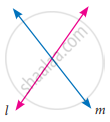•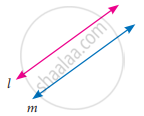•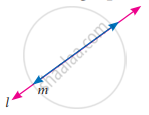•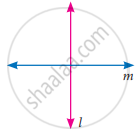Exercise 3.15 | Q 26 | Page 136

If "a"_1/"a"_2 ≠ "b"_1/"b"_2 where a1x + b1y + c1 = 0 and a2x + b2y + c2 = 0 then the given pair of linear equation has __________ solution(s)

• no solution

• two solutions

• unique

• infinite

Exercise 3.15 | Q 27 | Page 136

If "a"_1/"a"_2 = "b"_1/"b"_2 ≠ "c"_1/"c"_2 where a1x + b1y + c1 = 0 and a2x + b2y + c2 = 0 then the given pair of linear equation has __________ solution(s)

• no solution

• two solutions

• infinite

• unique

Exercise 3.15 | Q 28 | Page 136

G.C.D of any two prime numbers is __________

• −1

• 0

• 1

• 2

Exercise 3.15 | Q 29 | Page 136

The G.C.D of x4 – y4 and x2 – y2 is

• x4 – y4

• x2 – y2

• (x + y)2

• (x + y)4

Advertisement Remove all ads

## Chapter 3: Algebra

Exercise 3.1Exercise 3.2Exercise 3.3Exercise 3.4Exercise 3.5Exercise 3.6Exercise 3.7Exercise 3.8Exercise 3.9Exercise 3.10Exercise 3.11Exercise 3.12Exercise 3.13Exercise 3.14Exercise 3.15## Samacheer Kalvi solutions for Mathematics Class 9th Tamil Nadu State Board chapter 3 - Algebra

Samacheer Kalvi solutions for Mathematics Class 9th Tamil Nadu State Board chapter 3 (Algebra) include all questions with solution and detail explanation. This will clear students doubts about any question and improve application skills while preparing for board exams. The detailed, step-by-step solutions will help you understand the concepts better and clear your confusions, if any. Shaalaa.com has the Tamil Nadu Board of Secondary Education Mathematics Class 9th Tamil Nadu State Board solutions in a manner that help students grasp basic concepts better and faster.

Further, we at Shaalaa.com provide such solutions so that students can prepare for written exams. Samacheer Kalvi textbook solutions can be a core help for self-study and acts as a perfect self-help guidance for students.

Concepts covered in Mathematics Class 9th Tamil Nadu State Board chapter 3 Algebra are Concept of Polynomials, Algebraic Expressions, Value of a Polynomial, Roots of a Polynomial Equation, Concept of Identity, Polynomials in One Variable, Standard Form of a Polynomial, Degree of Polynomial, Remainder Theorem, Expansion of (a + b)2 = a2 + 2ab + b2, Expansion of (a - b)2 = a2 - 2ab + b2, Expansion of (a + b)(a - b), Types of Polynomials, Expansion of (x + a)(x + b), Arithmetic of Polynomials, Factor Theorem, Addition of Polynomials, Subtraction of Polynomials, Multiplication of Two Polynomials, Expansion of (a + b + c)2, Synthetic Division, Expansion of (x + a)(x + b)(x + c), Expansion of (a + b)3, Expansion of (a - b)3, Factorisation Using Identities, Highest Common Factor, Factorisation Using Identity a2 + 2ab + b2 = (a + b)2, Factorisation Using Identity a2 - 2ab + b2 = (a - b)2, Factorisation Using Identity a2 - b2 = (a + b)(a - b), General Form of Linear Equation in Two Variables, Factorisation using Identity a2 + b2 + c2 + 2ab + 2bc + 2ac = (a + b + c)2, Graph of a Linear Equation in Two Variables, Factorisation using Identity a3 + b3 = (a + b)(a2 - ab + b2), Factorisation using Identity a3 - b3 = (a - b)(a2 + ab + b2), Factorisation using Identity a3 + b3 + c3 - 3abc = (a + b + c)(a2 + b2 + c2 - ab - bc - ca), Factorising the Quadratic Polynomial (Trinomial) of the type ax2 + bx + c, a ≠ 0., Division Algorithm for Polynomials, Simultaneous Linear Equations, Methods of Solving Simultaneous Linear Equations by Graphical Method, Methods of Solving Simultaneous Linear Equations by Substitution, Methods of Solving Simultaneous Linear Equations by Cross Multiplication Method, Methods of Solving Simultaneous Linear Equations by Elimination Method, Comparing the Ratios of Coefficients of a Linear Equation, Consistency and Inconsistency of Linear Equations in Two Variables, Zeroes of a Polynomial.

Using Samacheer Kalvi Class 9th solutions Algebra exercise by students are an easy way to prepare for the exams, as they involve solutions arranged chapter-wise also page wise. The questions involved in Samacheer Kalvi Solutions are important questions that can be asked in the final exam. Maximum students of Tamil Nadu Board of Secondary Education Class 9th prefer Samacheer Kalvi Textbook Solutions to score more in exam.

Get the free view of chapter 3 Algebra Class 9th extra questions for Mathematics Class 9th Tamil Nadu State Board and can use Shaalaa.com to keep it handy for your exam preparation

Advertisement Remove all ads
Share
Notifications

View all notifications

Forgot password?